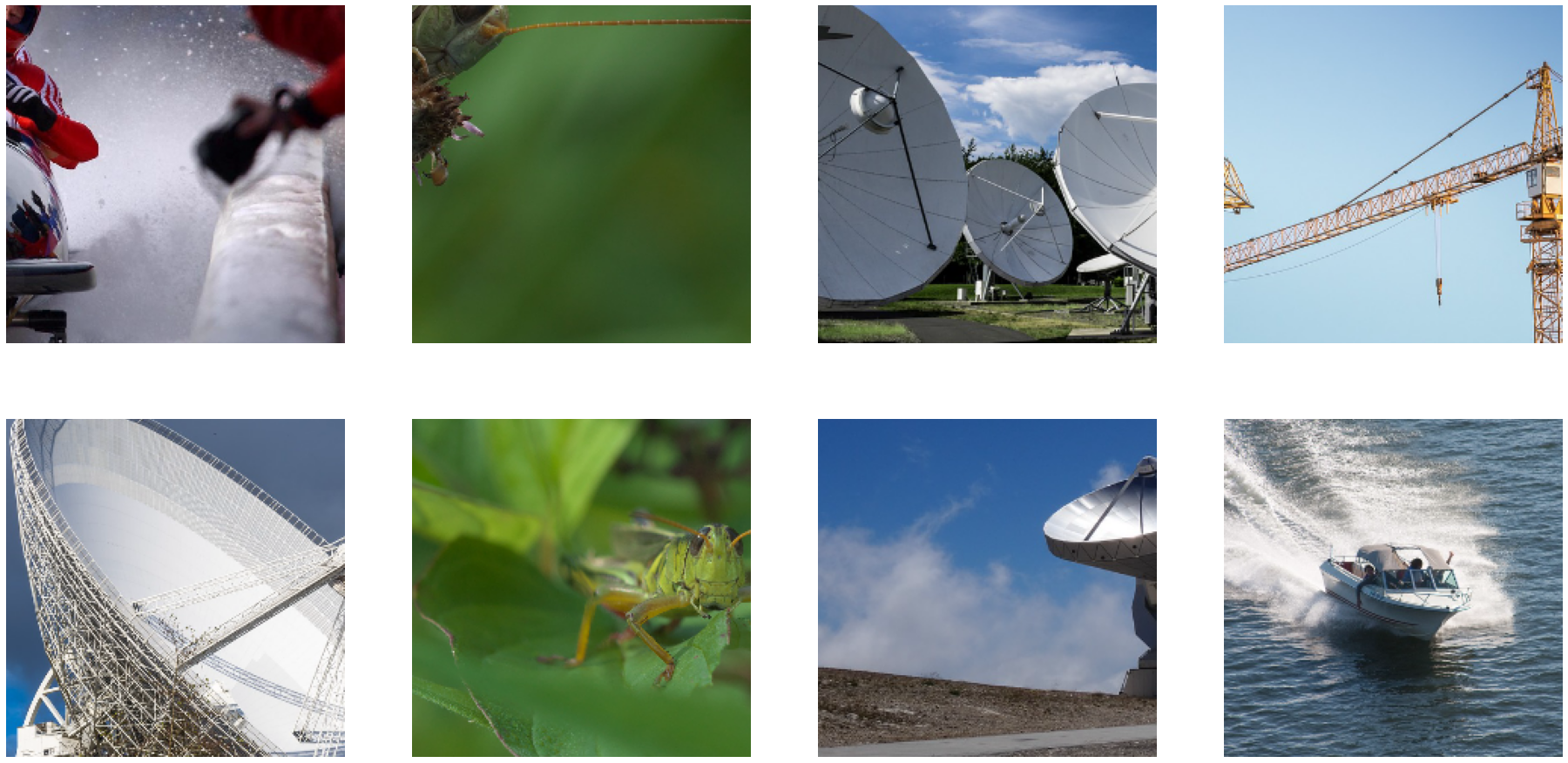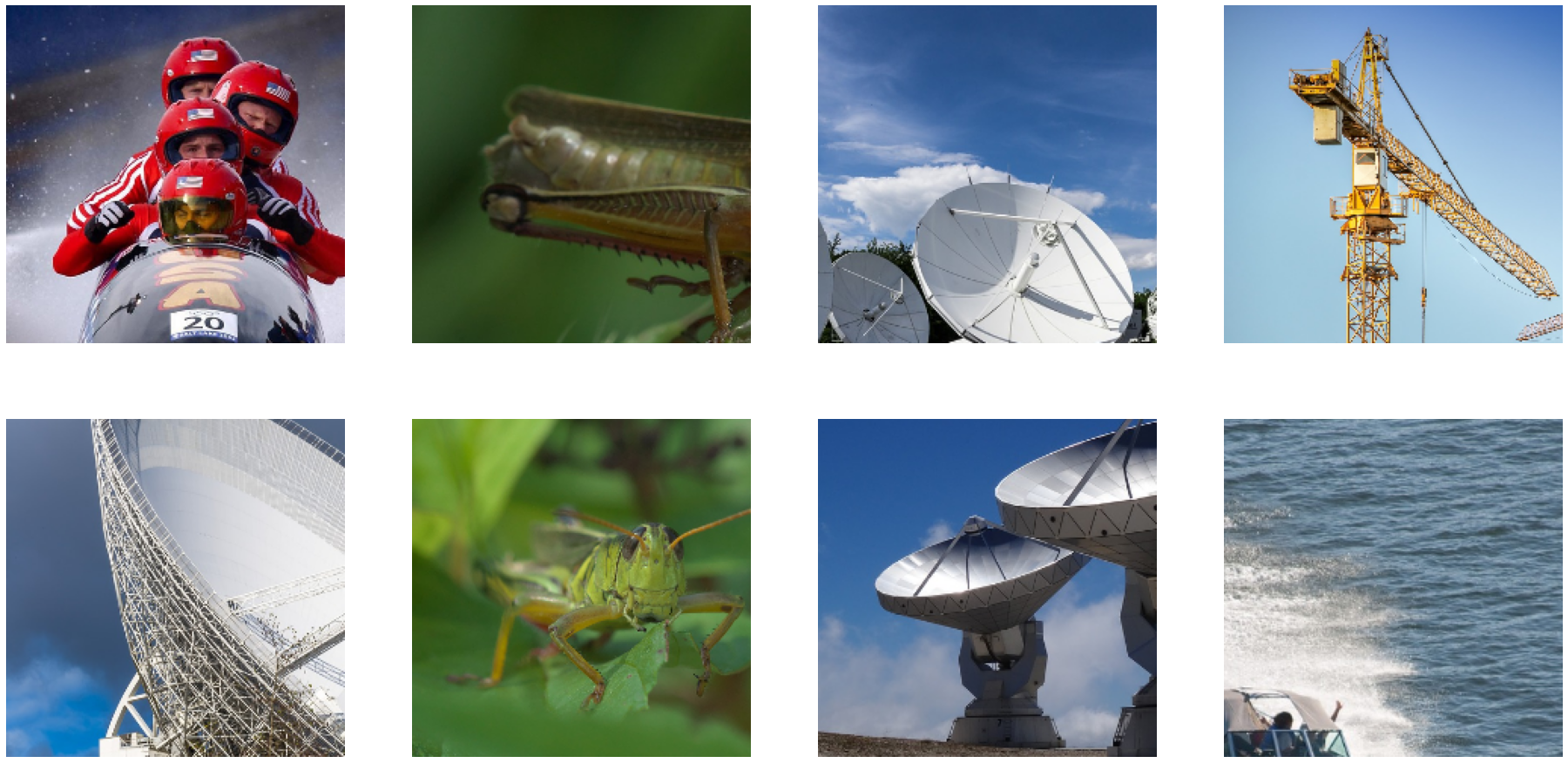# Using Tensorflow DALI plugin: simple example¶

## Overview¶

Using our DALI data loading and augmentation pipeline with Tensorflow is pretty simple.

We can summarize the integration in 3 steps :

• Defining a DALI Pipeline

• Give the pipeline to DALIIterator op

• Instatiate the op in TensorFlow graph and use it

First we start by defining some parameters for DALI and Tensorflow.

In this tutorial, we will use a subsample of Imagenet stored in an MXNet’s RecordIO. For details on how to use readers.mxnet, as well as other readers, please see other examples.

DALI_EXTRA_PATH environment variable should point to the place where data from DALI extra repository is downloaded. Please make sure that the proper release tag is checked out.

:

import os.path

test_data_root = os.environ['DALI_EXTRA_PATH']

# MXNet RecordIO
base = os.path.join(test_data_root, 'db', 'recordio')

idx_files = [base + "/train.idx"]
rec_files = [base + "/train.rec"]

BATCH_SIZE = 32
ITERATIONS = 32
BURNIN_STEPS = 16


In order to use DALI we need those 3 imports:

:

from nvidia.dali import pipeline_def, Pipeline
import nvidia.dali.fn as fn
import nvidia.dali.types as types


Then we can define our pipeline by declaring rn50_pipeline as as sublass of dali.pipeline.Pipeline. We declare the operators the pipeline will need in the constructor. Then we define the graph in define_graph.

Note again that we are using readers.mxnet that reads MXNet’s dataset format RecordIO. You can change it to other reader operators to use any of the supported dataset format.

:

@pipeline_def(batch_size=BATCH_SIZE, num_threads=4)
def rn50_pipeline(device):
images = fn.decoders.image(jpegs, device='mixed' if device == 'gpu' else 'cpu')
images = fn.resize(
images,
resize_shorter=fn.random.uniform(range=(256., 480.)),
interp_type=types.INTERP_LINEAR)
images = fn.crop_mirror_normalize(
images,
crop_pos_x=fn.random.uniform(range=(0.0, 1.0)),
crop_pos_y=fn.random.uniform(range=(0.0, 1.0)),
dtype=types.FLOAT,
crop=(224, 224),
mean=[128., 128., 128.],
std=[1., 1., 1.])
images = fn.cast(images, dtype=types.INT32)

if device == 'gpu':
labels = labels.gpu()

return images, labels


Next, we instatiate the pipelines with the right parameters. We will create one pipeline per GPU, by specifying the right device_id for each pipeline.

The difference is that instead of calling pipeline.build and using it, we will pass the pipeline object to the TensorFlow operator.

:

pipe = rn50_pipeline(device_id=0, device='gpu')


## Using DALI TensorFlow Plugin¶

Let’s start by importing Tensorflow and the DALI Tensorflow plugin as dali_tf.

:

import tensorflow as tf
import nvidia.dali.plugin.tf as dali_tf
import time

from tensorflow.compat.v1 import GPUOptions
from tensorflow.compat.v1 import ConfigProto
from tensorflow.compat.v1 import Session
from tensorflow.compat.v1 import placeholder

tf.compat.v1.disable_eager_execution()


We can now use nvidia.dali.plugin.tf.DALIIterator() method to get the Tensorflow Op that will produce the tensors we will use in the Tensorflow graph.

For each DALI pipeline, we use daliop that returns a Tensorflow tensor tuple that we will store in image, label. Each one is using a different tf.device.

:

daliop = dali_tf.DALIIterator()

images = []
labels = []
with tf.device('/gpu:0'):
image, label = daliop(
pipeline = pipe,
shapes = [(BATCH_SIZE, 3, 224, 224), ()],
dtypes = [tf.int32, tf.float32])

images.append(image)
labels.append(label)


## Using the Tensors in a Simple Tensorflow Graph¶

We will use images and labels tensors list in our Tensorflow graph definition. Then run a very simple one op graph session that will output the batch of images and labels.

:

gpu_options = GPUOptions(per_process_gpu_memory_fraction=0.8)
config = ConfigProto(gpu_options=gpu_options)

with Session(config=config) as sess:
all_img_per_sec = []
total_batch_size = BATCH_SIZE

for i in range(ITERATIONS):
start_time = time.time()

# The actual run with our dali_tf tensors
res = sess.run([images, labels])

elapsed_time = time.time() - start_time
img_per_sec = total_batch_size / elapsed_time
if i > BURNIN_STEPS:
all_img_per_sec.append(img_per_sec)
print("\t%7.1f img/s" %  img_per_sec)

print("Total average %7.1f img/s" % (sum(all_img_per_sec) / len(all_img_per_sec)))

         1807.8 img/s
1776.1 img/s
1926.8 img/s
1844.0 img/s
1787.0 img/s
1932.2 img/s
1792.4 img/s
1724.5 img/s
1436.6 img/s
1724.1 img/s
1711.2 img/s
1662.4 img/s
1694.4 img/s
1382.5 img/s
1423.9 img/s
Total average  1708.4 img/s


Let us check the output images with their augmentations! Tensorflow outputs numpy arrays, so we can visualize them easily with matplotlib.

We define a show_images helper function that will display a sample of our batch.

The batch layout is NCHW so we use transpose to get HWC images, that matplotlib can show.

:

import matplotlib.gridspec as gridspec
import matplotlib.pyplot as plt
%matplotlib inline

def show_images(image_batch, nb_images):
columns = 4
rows = (nb_images + 1) // (columns)
fig = plt.figure(figsize = (32,(32 // columns) * rows))
gs = gridspec.GridSpec(rows, columns)
for j in range(nb_images):
plt.subplot(gs[j])
plt.axis("off")
img = image_batch[j].transpose((1,2,0)) + 128
plt.imshow(img.astype('uint8'))
show_images(res, 8)Let us create the same operator for the CPU:

:

cpu_pipe = rn50_pipeline(device='cpu', device_id=0)

daliop = dali_tf.DALIIterator()

images_cpu = []
labels_cpu = []
with tf.device('/cpu'):
image_cpu, label_cpu = daliop(
pipeline=cpu_pipe,
shapes=[(BATCH_SIZE, 3, 224, 224), ()],
dtypes=[tf.int32, tf.float32])

images_cpu.append(image_cpu)
labels_cpu.append(label_cpu)

with Session() as sess:
all_img_per_sec = []
total_batch_size = BATCH_SIZE

for i in range(ITERATIONS):
start_time = time.time()

# The actual run with our dali_tf tensors
res_cpu = sess.run([images_cpu, labels_cpu])

elapsed_time = time.time() - start_time
img_per_sec = total_batch_size / elapsed_time
if i > BURNIN_STEPS:
all_img_per_sec.append(img_per_sec)
print("\t%7.1f img/s" %  img_per_sec)

print("Total average %7.1f img/s" % (sum(all_img_per_sec) / len(all_img_per_sec)))
show_images(res_cpu, 8)

          467.6 img/s
445.4 img/s
498.5 img/s
436.1 img/s
431.3 img/s
478.7 img/s
354.2 img/s
417.8 img/s
459.0 img/s
685.4 img/s
436.5 img/s
465.8 img/s
483.0 img/s
434.8 img/s
460.5 img/s
Total average   463.6 img/s[ ]: